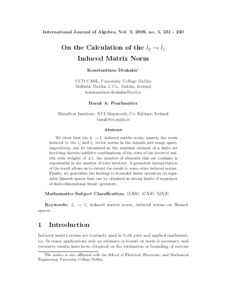# On the Calculation of the l2→l1 Induced Matrix Norm

Drakakis, Konstantinos and Pearlmutter, Barak A. (2009) On the Calculation of the l2→l1 Induced Matrix Norm. International Journal of Algebra, 3 (5). pp. 231-240. ISSN 1312-8868Previewmore...Add this article to your Mendeley library

## Abstract

We show that the l2 → l1 induced matrix norm, namely the norm induced by the l2 and l1 vector norms in the domain and range space, respectively, can be calculated as the maximal element of a finite set involving discrete additive combinations of the rows of the involved matrix with weights of ±1; the number of elements this set contains is exponential in the number of rows involved. A geometric interpretation of the result allows us to extend the result to some other induced norms. Finally, we generalize the findings to bounded linear operators on separable Banach spaces that can be obtained as strong limits of sequences of finite-dimensional linear operators.

Item Type: Article l2→l1 induced matrix norm, induced norms on Banach spaces Faculty of Science and Engineering > Research Institutes > Hamilton Institute 8176 Barak Pearlmutter 19 Nov 2018 17:54 International Journal of Algebra Hikari Yes Science Foundation Ireland (SFI)Item control page